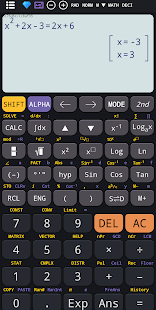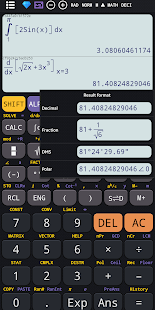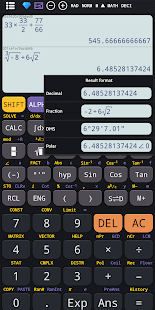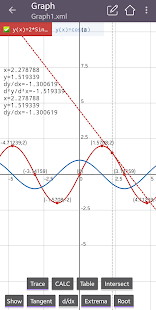Descriptions :

Scientific calculator 300 plus, 991 is the calculator for engineering students and students. Calculator 991 plus provides powerful functions on a real 991300 calculator. Makes 991 Advanced Calculator become the most useful calculator for college and school. 115 plus advanced calculator: combines hypercalculator and simple scientific calculation. This calculator is an advanced 991 multifunctional calculator with all functions in one application. Such as linear algebra, calculus, complex numbers, display of results in rectangular and polar coordinates, matrix and vector.

Required Android Versions : Jelly Bean [4.2–4.3.1] - KitKat [4.4–4.4.4] - Lollipop [5.0–5.0.2] - Marshmallow [6.0 - 6.0.1] - Nougat [7.0 – 7.1.1] - Oreo [8.0-8.1]